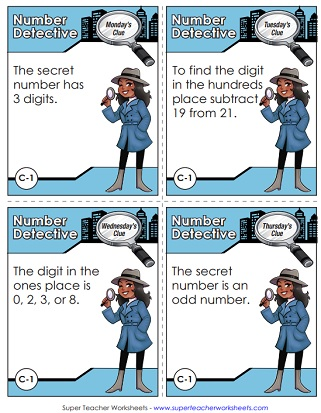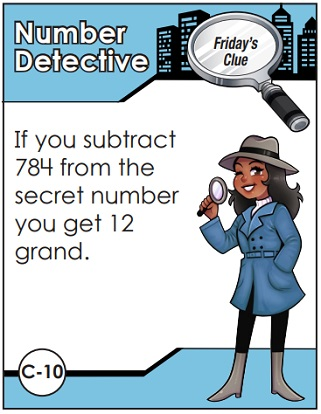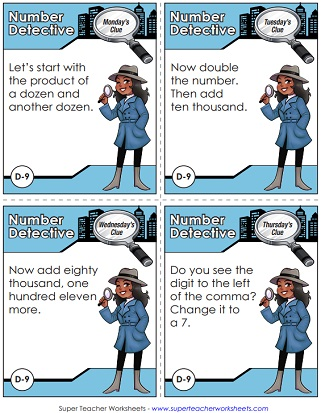# Number Detective

Post the daily Number Detective clues.  Students read the clue each day and try to narrow down the secret number.  These worksheets feature a cartoon detective and include daily cards, as well as answer cards that students can fill out when they solve the puzzle.The secret number is less than 100, but greater than 60.  It's an odd number with a seven in the tens place.  To find the number in the ones place, you add two and one.
The number you're looking for is less than thirty and it's not even.  Count by 5s and you'll say this number.  I have a quarter in my pocket -  that must be a clue.
Both the first and second digits are odd.  To find the first digit, subtract sixteen from nine.  Both digits are the same.
This week, we have a three-digit number.  It's less than one hundred fifty and if you add all of the digits you get the number three.  If you find the sum of seventy-none and twenty-three, you'll have the secret number.
We're back to a two-digit mystery number.  The digits all contain a zero, three, or six.  Count by 3s and the 12th number you say is the secret one.
The number for this week does not have two-digits.  It's not an odd number, and it is IV when you write it as a Roman numeral.If you know how many sides a trapezoid has, the number is easy to figure out.
The secret number is an even number with six letters in its name.  It is less than 20 but greater than 10.

The digit found in the ones place is an eight, three, two, or a zero.  If you know how many sides a hexagon has, finding the tens place will be easy.  To find the hundreds, subtract twenty-one minus nineteen.
The number in the thousands place can be found by calculating forty-nine less than fifty-three.  The tens and hundreds place are the same.  Use subtraction again to find the value of the ones.
This is the largest secret number so far.  It has one comma, with two digits to the left of the comma.  To find the first two digits, you need to know how many minutes are in a quarter hour.
There are more than two digits in this number, but also less than four.  In the ones place you'll see the largest possible digit.  The value of the digit in the tens place is equal to seventy.
The digits in this number are 1, 2, 9, and 9.  Immediately after the comma, you'll spot a couple of upside-down sixes.  The smallest digit can be found in the ones position.
Maybe the picture of the octopus will help you figure out the number in the tens position.  Two digits are even and one is odd.
Before the comma is a number that equals one dozen.  The digits one through five are each used once in this number.  In the hundreds place there's a digit that's one higher than two.
29,206.  That's the secret number... but the digits aren't in the right order.  One of the twos should be in the thousands place.  The other two is in the hundreds place.
Lucky sevens - there's a pair of them in the mystery number.  There's also a pair of unlucky thirteens in this four-digit number.
If you subtract 784 from the secret number, you'll get 12 grand.  If you add 216 to this number, you'll have thirteen thousand.

This mystery number is a seven-digit whole number.  To find the digit in the hundred thousands place, calculate the quotient of 50 and 10.  The hundreds, thousands, and millions digit are all the same.
This mystery number is not a whole number - it's a fraction.  The denominator is equal to the number of sides on an octagon.  Divide fifty-six by 8 to find the numerator.
This number is a decimal that's less than two, but greater than one.  If you round the secret number to the nearest whole number you get 2.  The digit in the tenths place is odd.
The mystery number for this week is a mixed number.  One part of this number might be considered unlucky.  The difference between 660 and 647 is the denominator.
This time you'll be searching for a number that is lower than zero.  It's twenty-three less than fifteen.
How many years are in a century?  How many degrees are in a right angle?  How many days in March?  How many days in a leap year?  Add the answers to find the number.
There's a kid who just turned 60 months old. His age in years is one of the digits in the mystery decimal number.
To calculate this mystery number you'll need to know the quotient of 300 and 50, as well as the definition of a unit fraction.
Start with the product of a dozen and a dozen, then double it. Then add ten thousand and then another eighty thousand.
Solve these mystery fraction problems, then add the fractions to find the mystery number.

## Blank Template

Make your own Number Detective clues with this blank template.  It includes the full page layout, the Monday through Friday clue cards, and two versions of the student answer cards.

## Also on Super Teacher Worksheets:

Add the numbers horizontally and vertically to complete the addition square puzzles.

Secret Code Math

On these SCM worksheets, students will decode addition, subtraction, multiplication, and division problems before solving.

## Sample Worksheet ImagesMy Account
Site Information